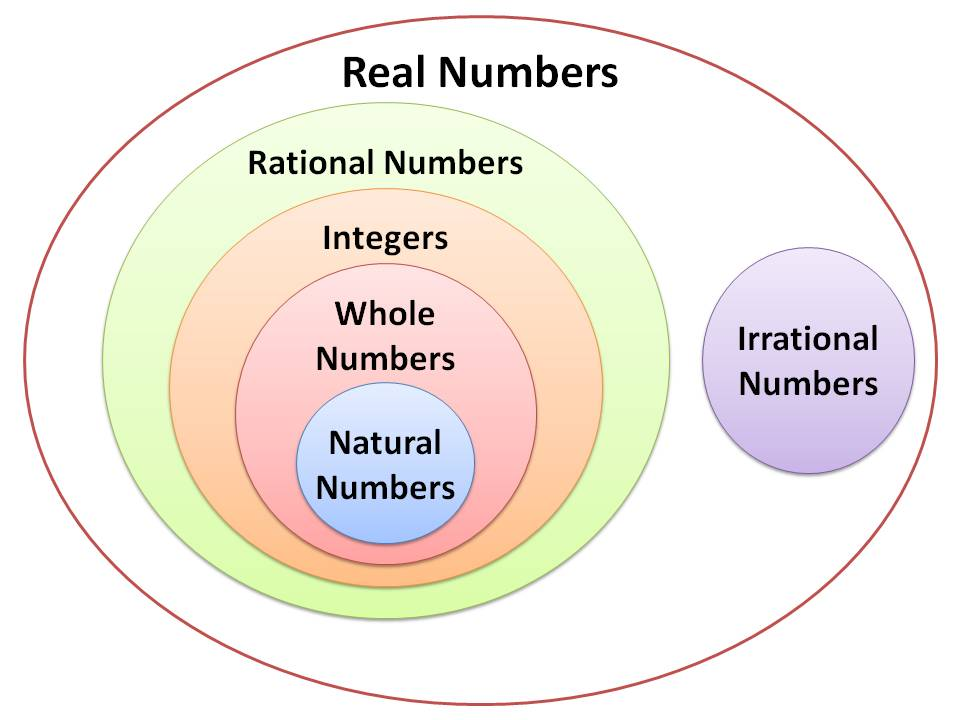# My Page of Learning -"Teaching the BEST Eagle Scholars in Mesa - 6th Grade!"

What are we learning in 6th grade?  In sixth grade we are learning about integers and rational numbers.

An integer is any whole number.  It is never a fraction.  My example of integers can be positive or negative numbers.Eample of the work we are doing:

Exercises
1. Scientists are studying temperatures and weather patterns in the Northern Hemisphere. They recorded
temperatures (in degrees Celsius) in the table below as reported in emails from various participants. Represent
each reported temperature using a rational number. Order the rational numbers from least to greatest. Explain
why the rational numbers that you chose appropriately represent the given temperatures.

1.

 MP.3

Scientists are studying temperatures and weather patterns in the Northern Hemisphere.  They recorded temperatures (in degrees Celsius) in the table below as reported in emails from various participants.  Represent each reported temperature using a rational number.  Order the rational numbers from least to greatest.  Explain why the rational numbers that you chose appropriately represent the given temperatures.

 Temperatures as Reported8 below zero 12413 below zero0 2 above zerobelow zeroTemperatureThe words “below zero refer to negative numbers because they are located below zero on a vertical number line.

Practice by playing this game:  Fun with Integers